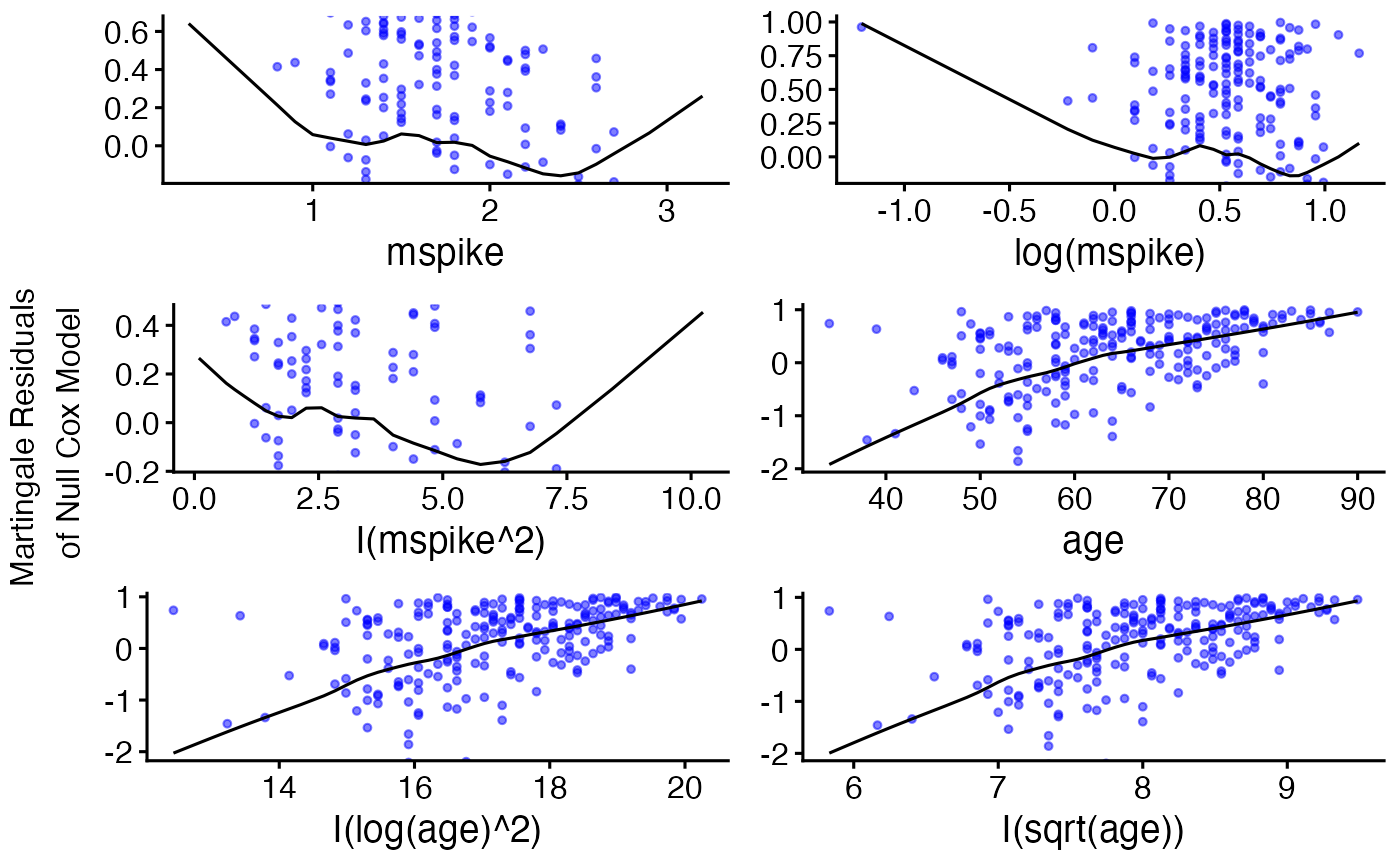Displays graphs of continuous explanatory variable against martingale residuals of null cox proportional hazards model, for each term in of the right side of formula. This might help to properly choose the functional form of continuous variable in cox model (coxph). Fitted lines with lowess function should be linear to satisfy cox proportional hazards model assumptions.

ggcoxfunctional(
formula,
data = NULL,
fit,
iter = 0,
f = 0.6,
point.col = "red",
point.size = 1,
point.shape = 19,
point.alpha = 1,
xlim = NULL,
ylim = NULL,
ylab = "Martingale Residuals \nof Null Cox Model",
title = NULL,
caption = NULL,
ggtheme = theme_survminer(),
...
)

# S3 method for ggcoxfunctional
print(x, ..., newpage = TRUE)

## Arguments

formula a formula object, with the response on the left of a ~ operator, and the terms on the right. The response must be a survival object as returned by the Surv function. a data.frame in which to interpret the variables named in the formula, an object of class coxph.object - created with coxph function. parameter of lowess. parameter of lowess. color, size, shape and visibility to be used for points. x and y axis limits e.g. xlim = c(0, 1000), ylim = c(0, 1). y axis label. the title of the final grob (top in arrangeGrob) the caption of the final grob (bottom in arrangeGrob) function, ggplot2 theme name. Allowed values include ggplot2 official themes: see theme. further arguments passed to the function ggpar for customizing the plot. an object of class ggcoxfunctional open a new page. See grid.arrange.

## Value

Returns an object of class ggcoxfunctional which is a list of ggplots.

## Functions

• ggcoxfunctional: Functional Form of Continuous Variable in Cox Proportional Hazards Model.

## Examples


library(survival)
data(mgus)
res.cox <- coxph(Surv(futime, death) ~ mspike + log(mspike) + I(mspike^2) +
age + I(log(age)^2) + I(sqrt(age)), data = mgus)
ggcoxfunctional(res.cox,  data = mgus, point.col = "blue", point.alpha = 0.5)ggcoxfunctional(res.cox, data = mgus, point.col = "blue", point.alpha = 0.5,
title = "Pass the title", caption = "Pass the caption")